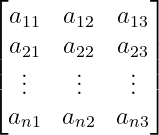# How to denote latex vertical dots(⋮) symbol?

Vertical dots are a symbol consisting of a combination of three dots along the vertical. With the help of this vertical dot symbol, I represent different mathematical expressions. Such as the matrix.

 Properties Value Symbol Vertical dots symbol Package Default Command \vdots Argument No Example \vdots →To represent latex vertical dots symbol, you need to use the \vdots command.

\documentclass{article}
\begin{document}
$$\vdots$$
\end{document}

Output :In matrix, when n numbers element are arranged along the row. In that case, vertical dots symbol is used instead of representing all the elements in the matrix along the vertical row.

\documentclass{article}
\usepackage{amsmath}
\begin{document}
$$\begin{bmatrix} a_{11}& a_{12} & a_{13} \\ a_{21}& a_{22} & a_{23} \\ \vdots & \vdots & \vdots \\ a_{n1} & a_{n2} & a_{n3} \end{bmatrix}$$
\end{document}

Output :To represent the matrix with latex, you first need to create a matrix environment. And then you have to insert the elements in the matrix.#### Md Jidan Mondal

LaTeX expert with over 10 years of experience in document preparation and typesetting. Specializes in creating professional documents, reports, and presentations using LaTeX.